# Time and Distance Quant Notes | EduRev

## Quant : Time and Distance Quant Notes | EduRev

The document Time and Distance Quant Notes | EduRev is a part of the Quant Course Quantitative Techniques for CLAT.
All you need of Quant at this link: Quant

Chapter - 16

TIME AND DISTANCE

FEW FORMULAE

Distance travelled = speed x time

Speed = distance / time

Time = distance / speed

• 1km/hr = (5/18) meter / sec
• 1 metre / sec = 18 / 5 km / hr.

If the time taken, to travel two distances with different speeds, is equal then

Average speed = (speed1 + speed 2) / 2

Also

average speed = total distance travelled  / total time taken

if two distances travelled are equal and two speeds are x km /hr and y km / hr

then average speed =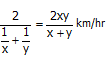if 3 equal distances are travelled at speeds x km /hr, ykm/ hr and z km/hr respectively then average speed =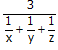FORMULAE FOR QUESTIONS ON TRAINS

•  To pass a pole or signal post or a standing man, a train has to travel a distance equal to its length.

So the time taken to pass a pole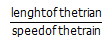• To pass a platform of length A, the train has to travel a distance equal to its length + the length of the platform (A)

So time taken by the train (length T) to pass a platform (length A) = T+A / Speed

• Let the speeds of two trains be x km / hr and y km /hr then

The relative speed if the direction of the trains is the same = (x – y) km/ hr

The relative speed if the direction of trains is opposite to each other

= (x+y) km /hr

SOLVED EXAMPLES

Example 1: A bike crosses a bridge at a speed of 180 km/hr. what will be length of the bridge if the bike takes 3 minutes to cross the bridge.

• Length of the bridge = distance travelled by the bike in 3 minutes = speed x time

Speed = 180 km/hr = (180 x 5) / 18 = 50 meters / sec.

Time = 3 x 60 = 180 seconds.

So length of the bridge

50 X 180 meters

= (50 x 180) / 1000 km

= 9 km

Ex. 2: A person covers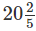km in 6 hours. What distance will he cover in 5 hours.

• speed of the person = distance / time =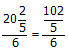= 102/30 km/hr

Distance covered in 5 hours = (102/30) x 5 = 17 km

Ex. 3: Two persons are moving in the direction opposite to each other. The speeds of the two persons are 8 km/hr and 5 km per hour. Find their relative speed wrt each other

• We know that when two objects move in the direction opposite to each other, the relative speed is the sum of two speeds.

So required relative speed = 8 + 5 = 13 km/hr

Ex. 4: A bus covers a distance of 600 km with a speed of 30 km/hr. How much time will it take to cover the distance.

• Time taken = Distance / Speed

= 600 / 30 = 20 hours

Ex. 5: Two trains A and B are moving at the speeds in the ratio of 2 : 5. Find the ratio of the time taken to travel the same distance.

• The ratio of time taken is inverse of the speeds of the two cars.

i.e. since speeds are 2 : 5

so time must be 5 : 2

Ex. 6: Prem can cover a certain distance in 42 minutes by covering 2/3 of the distance at 4 km/hr and the rest at 5 km/hr. find the total distance.

• Let total distance be x

So as per the given condition.

Distance / speed + distance / speed = total time

Or (2x/3) x ¼ + (x/3) x (1/5) = 42/60

Or x/2 + x/5 = 42/20

7x/10 x= 42/20

X = 3 km

Example 7: A man completes 60 km of a journey at 12 km / hr and the remaining 80 km of the journey in 5 hours. Find the average speed for the whole journey.

• Total distance travelled = 60 km + 80 km = 140 km

Total time taken = 5 hours + 5 hours = 10 hours

So average speed for the whole journey = 140/10

= 14 km/hr

1.  Speed during the first part = 12 km/hr

Time taken during the first part to cover 60 km = 60 / 12= 5 hours

Time taken to cover the second part = 5 hrs

And the speed during 2nd part = 80/5 = 16 km/hr

So the time taken during the two journeys is equal

So average speed = (12 km/hr + 16 km/hr) / 2 = 28/2

= 14 km/hr

Ex 8: A certain distance is covered at a certain speed. If half the distance is covered in double the time, the ratio of two speeds is

• Let the distance covered be x and the speed be S  and time taken be T. then

S = X / T

Now in the 2nd case distance is x/2

And time is = 2 T

The speed is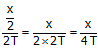The ratio in the speed is X/T = X / 4T

Or 1 : ¼ or 4 : 1

Ex. 9: A bullock cart has to cover a distance of 40 km in 5 hours. If it covers half of the journey in 3/5 th of the time, what should be its speed to cover the remaining distance in the left over time.

• 40 kms are to be covered in 5 hours 20 kms are covered in 3/5 x 5 i.e. 3 hours. In the left over 2 hours 20 kms are to be covered. So the speed must be

20/2 = 10 km/hr

Ex. 10: A car travels from A to B at a speed of 58 km/hr and travels back from B to A at the speed of 42 km/hr. what is average speed of the car in covering the distance both ways.

• Since the car travels equal distance with different speed, the average speed is the harmonic mean of the two speeds.

Average speed =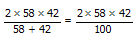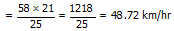Ex. 11: The speed of A and B are in the ratio 3 : 4. A takes 20 minutes more than the time taken by B to reach a destination. In what time doesA reach the destination

• Let time taken by A be x hours.

Then time taken by B = (x – 20/60) hours

Or (x – 1/3) hours

Ratio of speeds = inverse ratio of time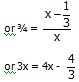or x = 4/3 hours

Example 12: A train 100 meter long is running at a speed of 30 km/hr. Find the time taken by the train to pass a man standing near the railway line.

• Speed of the train = 30 km/hr = (30 x 5)/18 meter / sec.

Distance to be travelled to pass the man = 100 meter

So time to pass the man = distance / speed =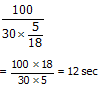Ex. 13: A train is moving at a speed of 66 km/hr. if the length of the train is 55 metre, how long will it take to cross a plat form 165 meter long.

• Speed of the train = 66 km/hr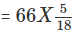meter / sec

Distance to be covered in crossing the palt form

= 55 + 165 = 220 meter.

Time required = (220) / (55 /3)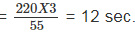Ex. 14: A train crosses a platform in 20 seconds but a man standing on the platform in 8 seconds. Length of the platform is 180 meters. Find the length of the train and its speed

• Time taken to cross the platform = 20 sec.

Time taken to cross the man = 8 sec.

20 – 8 = 12 sec is the time taken by the train to travel the distance equal to platform.

The train travels 180 meter in 12 seconds

So its speed is 180/12 = 15 m/sec.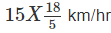And the length of the train = distance travelled in

8 sec. = 15 x 8 = 120 meter

Example 15: A train 200 m long is running at a speed of 68 km/hr. in what time will it pass a man who is running at 8 km/hr in the same direction in which train is going

• The relative speed of the train = 68 – 8 = 60 km/hr

To cross the man the train has to travel a distance equal to its length

60 km/hr = (60 x 5)/18 m/ sec. = 300/ 18 meter/ sec.

So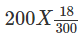= 12 minutes

Ex. 16: A train 165 meter in length is running with a speed of 59 km/hr. in what time will it pass a man who is running at a speed of 7 km/hr in the direction opposite to that in which the train is going?

• The relative speed of the train wrt man = 59 + 7 = 66 km/hr.

Or (66 x 5)/18 meter / sec.

The time taken by the train to cross the man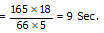Ex. 17: Two trains are running towards each other at the rate of 42 km/hr and 30 km/hr. The length of the trains is 120 meter and 80 meters respectively. In what time will they cross each other from the moment they meet.

• Relative speed of the trains = (42 + 30) km/hr

= 72 km/hr

= 72 x 5/18 = 20 m / sec

Time taken by the trains to pass each other = sum of their lengths divided by the relative speed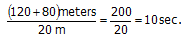Ex. 18: Two trains 100 meters and 80 meters long are running in the same direction with speeds 90 km/hr and 54 km/hr respectively. In how much time will the first train cross the second train.

• Relative speed of two trains = (90-54) km/hr

= 36 km/h = 36 x 5/18 = 10 meter / second

Time taken by the trains to cross each other

=sum of their lengths / relative speed

= (100+80) / 10 = 180/10 = 18 sec

Ex. 19: A train 100 meters long takes 6 seconds to cross a man walking at 10 km/hr in a direction opposite to that of the train. Find the speed of the train.

• Let the speed of the train be x km/hr. so relative speed of the train = (x + 10) km/hr

= (x + 10) x (5/18) meter / second

Now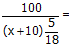6 seconds

Or 100 = 6 x (x + 10) x 5/18 = 5/3 (x + 10)

Or x + 10 = (100 x 3) / 5 = 60 or x = 50 km/hr

Ex. 20: A train running at 54 km/hr. takes 25 seconds to pass a platform and 15 seconds to pass a man walking at 6 km/h in the same direction in which train is going. Find the length of the train and the length of the platform.

• Let x and y be the length of the train and of the platform respectively.

Speed of the train w.r.t the man = 54 – 6 = 48 km/ph

= 48 x 5/18 = 40/3 meter / seconds

In passing the man the train covers its own length with the relative speed.

So the length of the train = Relative speed x time

= (40/3 x 15) m = 200 m.

Speed of the train = 54 x 5/18 = 15 m/second.

Distance travelled to pass the platform = x + y

So x + y = 200 + y

So (200 + y) / 15 = 25

Or y + 200 = 375

Or y = 175 meters.

Offer running on EduRev: Apply code STAYHOME200 to get INR 200 off on our premium plan EduRev Infinity!

## Quantitative Techniques for CLAT

61 videos|22 docs|98 tests

,

,

,

,

,

,

,

,

,

,

,

,

,

,

,

,

,

,

,

,

,

;